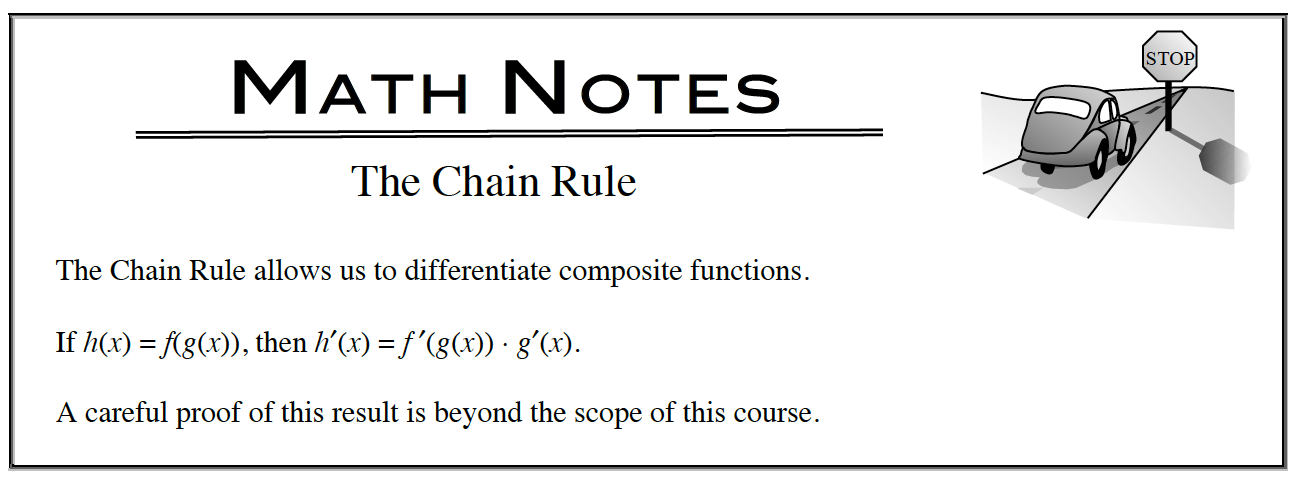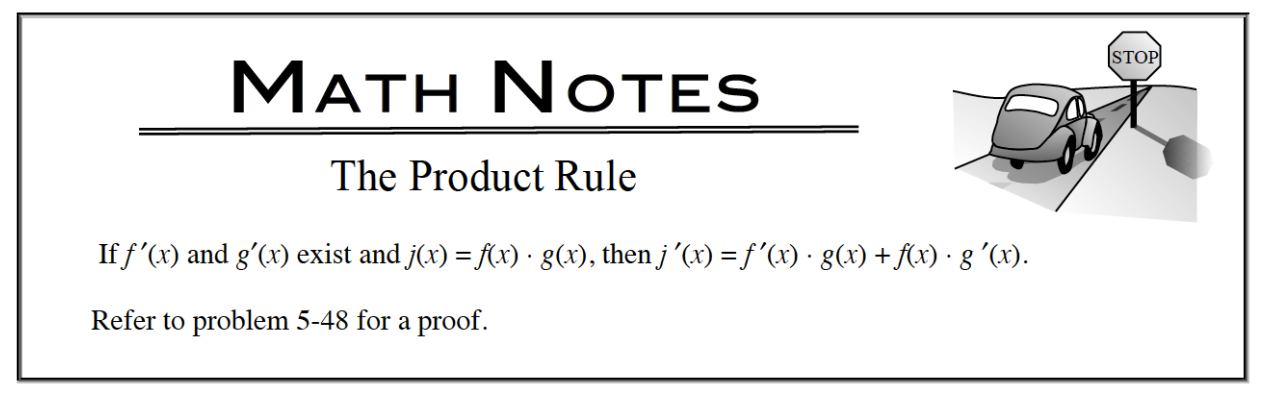### Home > APCALC > Chapter 5 > Lesson 5.2.3 > Problem5-80

5-80.

For parts (a) and (b) below, explain why obtaining the derivative requires the use of the Chain Rule and the Product Rule. Then write the derivative. Homework Help ✎

1. $\frac { d } { d x } \sqrt { \operatorname { sin } ( x ) \operatorname { cos } ( x ) }$

This derivative requires BOTH the Chain Rule and the Product Rule.

2. $\frac { d } { d x } ( \sqrt { x } \operatorname { sin } ( x ^ { 3 } ) )$

This derivative also requires the Chain Rule and the Product Rule, but in the opposite order as part (a).